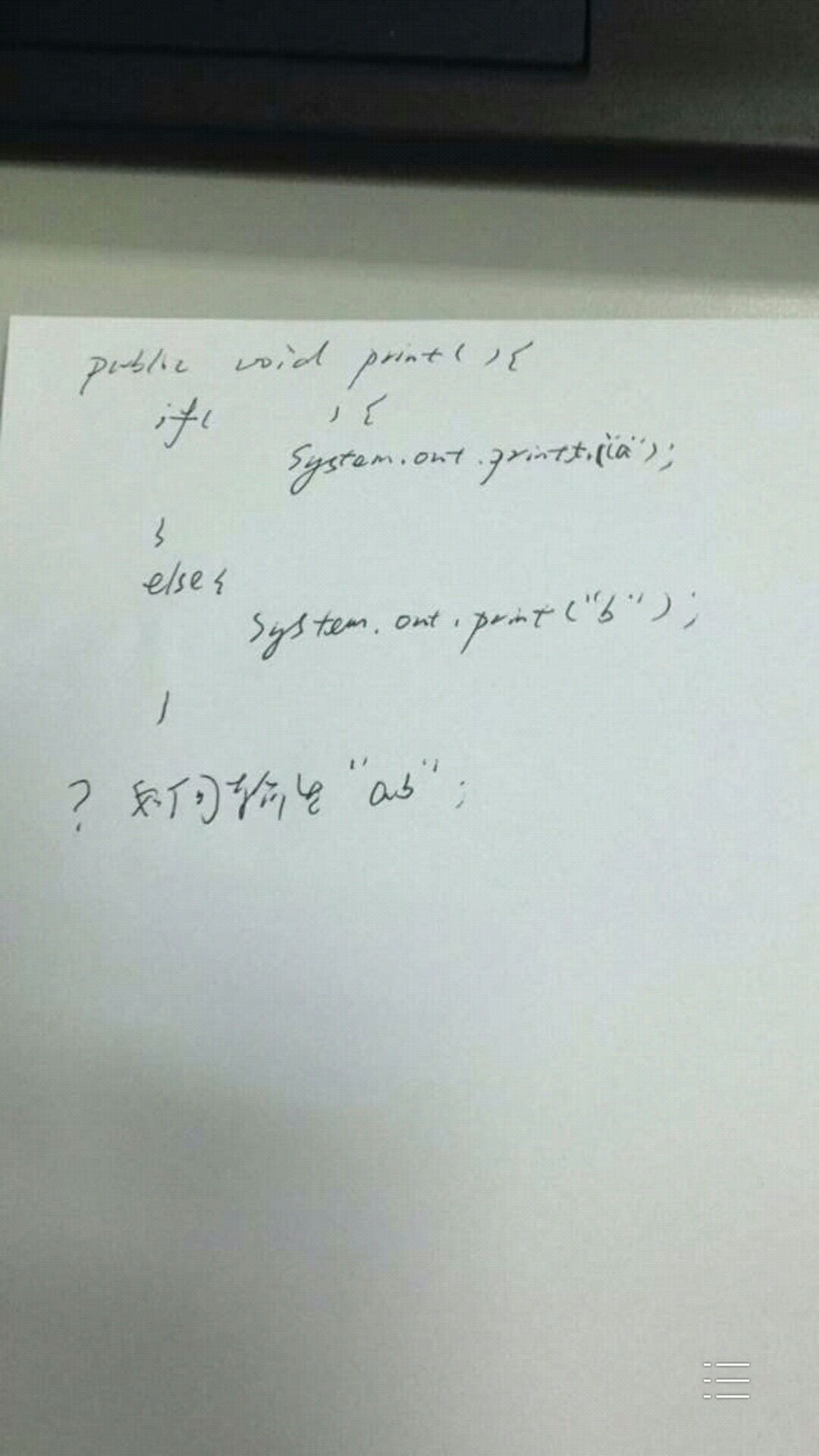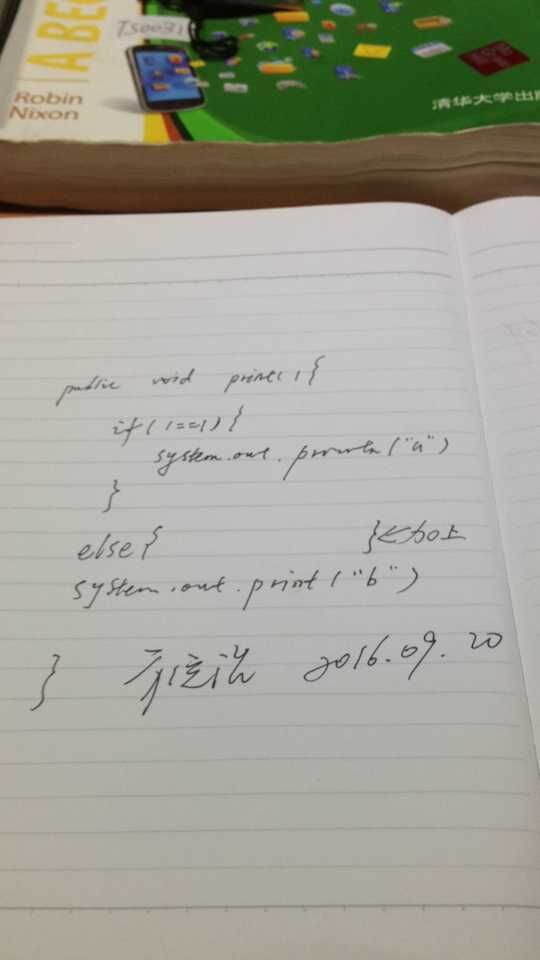Java

1人收藏 2488次学习

一道在知乎很火的 Java 题——如何输出 ab题目思路一

``````public void print() {
if (true) {
System.out.print("a");
}

if (false) {
System.out.print("a");
} else {
System.out.print("b");
}
}``````

思路二``````public void print() {
if (System.out.printf("a") == null) {
System.out.print("a");
} else {
System.out.print("b");
}
}``````

解法三

``````public void print() {
if (new Object() {
boolean print() {
System.out.print("a");
return false;
}
}.print()) {
System.out.print("a");
} else {
System.out.print("b");
}
}``````

解法四

``````public void print() {
if (((BooleanSupplier)(() -> {System.out.print("a");return false;})).getAsBoolean()) {
System.out.print("a");
} else {
System.out.print("b");
}
}``````

``import java.util.function.BooleanSupplier;``

脑洞大开

``````public void print() {
//    if ( ) {
System.out.print("a");
//    } else {
System.out.print("b");
//    }
}``````加入1KE学习俱乐部

1KE学习俱乐部是只针对1KE学员开放的私人俱乐部